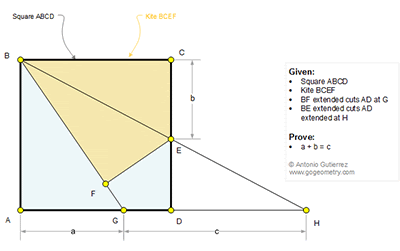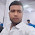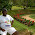## Sunday, April 30, 2017

### Geometry Problem 1334. Square, Kite, Sum of Segments

Geometry Problem. Post your solution in the comment box below.
Level: Mathematics Education, High School, Honors Geometry, College.

Details: Click on the figure below.1.Problem 1334
Is <GBE=<EBC=<EHG then GH=BG. Draw BK perpendicular in BE at point B (K belongs to ΑD).But triangle KBA=triangle BCE (rectangular, AB=BC,<KBA=<EBC) so KA=CE.Now rectangular triangle KBH is <KBG+<GBH=90=<BKH+<BHK so <KBG=<BKG ,then KG=BG=GH. Therefore GH=KG=KA+AG=CF+AG.
APOSTOLIS MANOLOUDIS 4 HIGH SCHOOL OF KORYDALLOS PIRAEUS GREECE

2.∆EDH ~ ∆BCE => x²= ab + bc ( x = AD )
From ∆ABG => x² = c² - a²
From two rows c = a + b

1.Excellent solution

2.Can you please explain the first line?

3.EC/BC=DE/DH, EC=b, BC=x, DE=x-b, DH=c-(x-a)

3.Extend DA to X such that AX = b.
Then Tr.s BCE,BEF & AXB are all congruent SAS and so < ABX = < GBH = < CBE < GHB.

Since AB is perpendicular to XH, it follows that < XBH = 90

But GB = GH so each of these = XG
Therefore a+b =c

Sumith Peiris
Moratuwa
Sri Lanka

4.Solution 2

Let AB = p

From similar triangles b/p = p/(a+c)
So p^2 = b(a+c) = c^2 - a^2 and hence dividing by a+c, b = c-a

Sumith Peiris
Moratuwa
Sri Lanka

5.Further result < AFC = 135

6.Draw BK ⊥ BE, K on DA. Draw circle through H, B, K
G center of the cirle

7.Triangle GBH is Isosceles and GB=a+b (One way to derive is considering the right triangle BFE and applying pythogorus)
=> c=a+b

8.Let length of square be 1.

<CBE=<EHD
<CBE= tan(-1)b
<EHD= tan(-1){1÷(a+c)}
So, b(a+c)=1

BG=GH
SQRT(1+a^2)=c (∆BAG)
By substituting b(a+c)=1 into SQRT(1+a^2)=c, you get a+b=c.